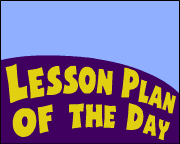## Search form# Beanbag Math

Subjects

Mathematics
--Arithmetic

K-2
3-5

Brief Description

Use this simple game to reinforce math facts and other math skills.

Objectives

Students will correctly solve math facts or other simple math equations.

Keywords

beanbag, math facts, multiplication tables

• chalk (for drawing grids on playground pavement/sidewalk areas)
• beanbags or another object (e.g., small stones, small sticks) to toss at the grid

Lesson Plan

This activity can be used to reinforce a wide variety of math skills. It is a fun activity to take outdoors. Use chalk to create the game grid on safe pavement or sidewalk areas (just as you might create a hopscotch grid there).

For the purpose of this explanation, we will use the game to reinforce the multiplication tables 1 to 5, but you could easily adapt the activity to reinforce multiplication facts to 10, addition facts, or subtraction facts. Or you might use random numbers on the grid (e.g., 17, 29, 14, 31, 18) to reinforce adding two 2-digit numbers.

Use chalk to create a grid on the floor or outdoor play surface. You might create a handful of grids so small groups of students can play together.Provide each child with a beanbag to toss. (Alternative: students might toss a small stone, stick, or another object.) Where does the beanbag land when tossed? If it lands in a square that is in the 3rd row and 4th column of the grid, students make a multiplication equation using those two numbers (3 x 4 = ) and solve the equation. Classmates will tell the student if the answer s/he gives is correct.

Alternate players and continue playing in this manner.

Adapt the game. Instead of using a 5 x 5 grid, create a 10 x 10 grid to reinforce the 1 to 10 multiplication tables.

Assessment

Classmates will identify whether or not students correctly solve equations. If a student solves incorrectly, classmates will give the student another opportunity to provide the correct response.

Lesson Plan Source

EducationWorld.com

Submitted By

Gary Hopkins

National Standards

MATHEMATICS: Number and Operations
NM-NUM.PK-2.3 Compute Fluently and Make Reasonable Estimates
NM-NUM.3-5.3 Compute Fluently and Make Reasonable Estimates

## More Lesson Ideas

Find links to more math lesson ideas in these Education World archives:

• Math Subject Center
• Daily Lesson Plans -- Math
• Lesson Planning/Math Archive
• Curriculum/Math Archive
• Math Subject Center
• Teacher-Submitted Lessons -- Math
• Number-Cross Puzzles (Printable Work Sheets)
• It All Adds Up Puzzles (Printable Work Sheets)
• More Math Printable Work Sheets
• Morning Math
• Calculator Lessons from Texas Instruments
• Pi Day (March 14)
• Special Days & Holidays Lessons
• Technology Lessons

Education World®